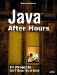Getting the Pucks to Bounce Off Each Other

 In the Aquarium application, one fish could swim behind another, but that doesn't work for multiple pucks on the same surface; instead, they're going to bounce off each other. Now that the pucks have all been moved, the run method works through them all to see if any pucks have hit each other. If so, it bounces them (and after the pucks have bounced, the run method checks again to see if they've hit a goal). Here's how the code checks whether one puck has struck another, taking special care not to move a blocker (that is, a Puck object whose immovable method returns TRue) if it was hit: int struckPuck = -1; for (int loopIndex3 = 0; loopIndex3 < 13; loopIndex3++){ Puck testPuck = (Puck)pucks.elementAt (loopIndex3); if (puck == testPuck || testPuck.gone()){ continue; } if(puck.rectangle.intersects (testPuck.rectangle)){ struckPuck = loopIndex3; } } if (struckPuck >= 0){ Puck puck1 = (Puck)pucks.elementAt(struckPuck); Puck puck2 = (Puck)pucks.elementAt(loopIndex); if(puck2.immovable()){ puck1.velocity.x = -puck1.velocity.x; retVal = puck1.slide(pucks.elementAt (13).rectangle, pucks.elementAt (12).rectangle); numberLeft = 0; for (int loopIndex4 = 0; loopIndex4 < 12; loopIndex4++){ if(!((Puck)pucks.elementAt (loopIndex4)).gone()){ numberLeft++; } } if(retVal < 0){ if(yourScore + theirScore + numberLeft == 11){ label1.setText(String.valueOf (++yourScore)); } } if(retVal > 0){ if(yourScore + theirScore + numberLeft == 11){ label2.setText(String.valueOf (++theirScore)); } } } else if(puck1.immovable()){ puck2.velocity.x = -puck2.velocity.x; retVal = puck2.slide(pucks.elementAt(13). rectangle, pucks.elementAt (12).rectangle); numberLeft = 0; for (int loopIndex5 = 0; loopIndex5 < 12; loopIndex5++){ if(!((Puck)pucks.elementAt (loopIndex5)).gone()){ numberLeft++; } } if(retVal < 0){ if(yourScore + theirScore + numberLeft == 11){ label1.setText(String.valueOf (++yourScore)); } } if(retVal > 0){ if(yourScore + theirScore + numberLeft == 11){ label2.setText(String.valueOf (++theirScore)); } } } else { retVal = puck1.slide(pucks.elementAt (13).rectangle, pucks.elementAt (12).rectangle); numberLeft = 0; for (int loopIndex6 = 0; loopIndex6 < 12; loopIndex6++){ if(!((Puck)pucks.elementAt(loopIndex6)) .gone()){ numberLeft++; } } if(retVal < 0){ if(yourScore + theirScore + numberLeft == 11){ label1.setText(String.valueOf (++yourScore)); } } if(retVal > 0){ if(yourScore + theirScore + numberLeft == 11){ label2.setText(String.valueOf (++theirScore)); } } retVal = puck2.slide(pucks.elementAt (13).rectangle, pucks.elementAt (12).rectangle); numberLeft = 0; for (int loopIndex7 = 0; loopIndex7 < 12; loopIndex7++){ if(!((Puck)pucks.elementAt (loopIndex7)).gone()){ numberLeft++; } } if(retVal < 0){ if(yourScore + theirScore + numberLeft == 11){ label1.setText(String.valueOf (++yourScore)); } } if(retVal > 0){ if(yourScore + theirScore + numberLeft == 11){ label2.setText(String.valueOf (++theirScore)); } } } } This code moves all the pucks around each time the all-encompassing while loop iterates. But what about the blockers? How do they move? To start, the computer moves its own blocker around by itself.Java After Hours: 10 Projects Youll Never Do at Work
ISBN: 0672327473
EAN: 2147483647
Year: 2006
Pages: 128

Similar book on Amazon